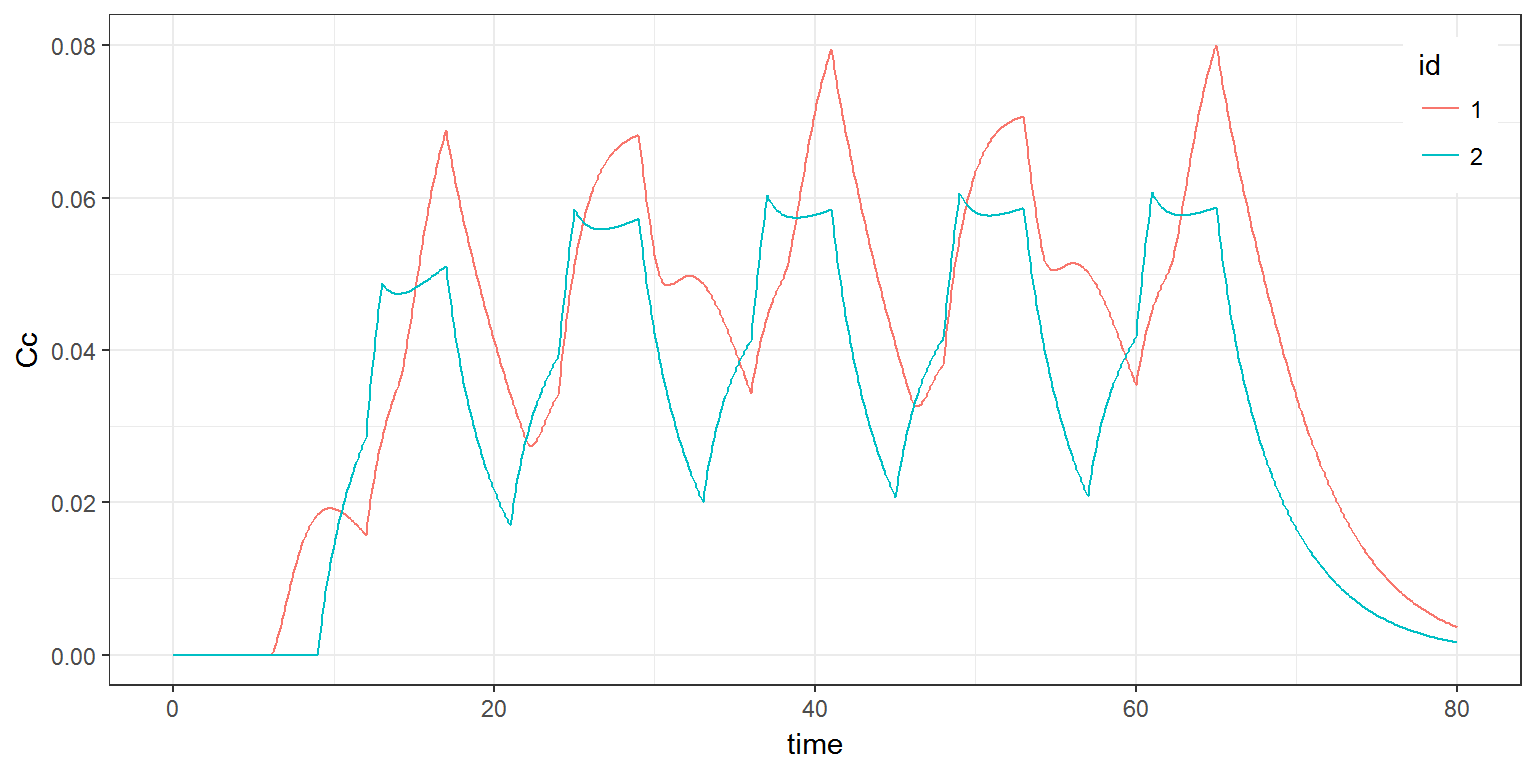R script: pk2.R

Mlxtran code: model/pk2a.txt ; model/pk2b.txt ; model/pk3.txt

$$\newcommand{\esp}{\mathbb{E}\left(#1\right)} \newcommand{\var}{\mbox{Var}\left(#1\right)} \newcommand{\deriv}{\dot{#1}(t)} \newcommand{\prob}{ \mathbb{P}\!(#1)} \newcommand{\eqdef}{\mathop{=}\limits^{\mathrm{def}}} \newcommand{\by}{\boldsymbol{y}} \newcommand{\bc}{\boldsymbol{c}} \newcommand{\bpsi}{\boldsymbol{\psi}} \def\pmacro{\texttt{p}} \def\like{{\cal L}} \def\llike{{\cal LL}} \def\logit{{\rm logit}} \def\probit{{\rm probit}} \def\one{{\rm 1\!I}} \def\iid{\mathop{\sim}_{\rm i.i.d.}} \def\simh0{\mathop{\sim}_{H_0}} \def\df{\texttt{df}} \def\res{e} \def\xomega{x} \newcommand{\argmin}{{\rm arg}\min_{#1}} \newcommand{\argmax}{{\rm arg}\max_{#1}} \newcommand{\Rset}{\mbox{\mathbb{R}}} \def\param{\theta} \def\setparam{\Theta} \def\xnew{x_{\rm new}} \def\fnew{f_{\rm new}} \def\ynew{y_{\rm new}} \def\nnew{n_{\rm new}} \def\enew{e_{\rm new}} \def\Xnew{X_{\rm new}} \def\hfnew{\widehat{\fnew}} \def\degree{m} \def\nbeta{d} \newcommand{\limite}{\mathop{\longrightarrow}\limits_{#1}} \def\ka{k{a}} \def\ska{k{\scriptscriptstyle a}} \def\kel{k{e}} \def\skel{k{\scriptscriptstyle e}} \def\cl{C{\small l}} \def\Tlag{T\hspace{-0.1em}{lag}} \def\sTlag{T\hspace{-0.07em}{\scriptscriptstyle lag}} \def\Tk{T\hspace{-0.1em}{k0}} \def\sTk{T\hspace{-0.07em}{\scriptscriptstyle k0}} \def\thalf{t{1/2}} \newcommand{\Dphi}{\partial_\pphi #1} \def\asigma{a} \def\pphi{\psi} \newcommand{\stheta}{{\theta^\star}} \newcommand{\htheta}{{\widehat{\theta}}}$$

# 1 Introduction

It is possible to define elementary administrations and then define a treatment as a combination of these elementary administrations.

# 2 Examples

## 2.1 Example 1

Assume now that we combine oral and iv administrations of the same drug.

We assume here a one compartment model with first-order absorption process from the depot compartment (oral administration) and a linear elimination process from the central compartment. We further assume that only a fraction $$F$$ (bioavailability) of the drug orally administered is absorbed.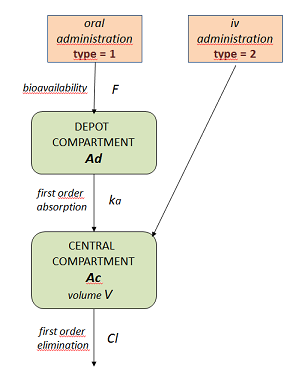Let $$A_d$$ and $$A_c$$ be, respectively, the amounts in the depot compartment (gut) and the central compartment (bloodtsream). Kinetics of $$A_d$$ and $$A_c$$ are described by the following system of ODEs \begin{aligned} \dot{A_d}(t) & = - ka \, A_d(t) \\\ \dot{A_c}(t) & = ka \, A_d(t) - k \, A_c(t) \end{aligned} The concentration $$C_c$$ in the central compartment is defined by $$\ \ C_c(t) = A_c(t)/V$$

The target compartment is the depot compartment ($$A_d$$) for oral administrations and the central compartment ($$A_c$$) for iv administrations. This model is implemented in pk2a.txt using a system of ODEs

[LONGITUDINAL]
input = {F, ka, V, k}

PK:
depot(type=1, target=Ad, p=F)
depot(type=2, target=Ac)

EQUATION:
ddt_Ad = -ka*Ad
ddt_Ac =  ka*Ad - k*Ac
Cc = Ac/V


Remark: the same model could be efficiently implemented using PK macros instead of ODEs (indeed, the system here is linear and there is no need to sove ODE systems when analytical solutions are available)

[LONGITUDINAL]
input = {F, ka, V, k}

PK:
compartment(cmt=1, amount=Ac)
oral(type=1, cmt=1, ka, p=F)
iv(type=2, cmt=1)
elimination(cmt=1, k)
Cc = Ac/V


Here, list adm1 defines the oral administrations. Then, an aditional field type=1 is used to link this administration with the PK model defined in the Mlxtran code.

List adm2 defines the iv infusion administrations with type=2.

When each elementary administration has been defined, treatment is defined as a list that includes all the elementary administrations.

adm1 <- list(type   = 1,
time   = c(6, 30, 36),
amount = 40)

adm2 <- list(type   = 2,
time   = c(12,42),
amount = 20,
rate   = c(5, 10))

p    <- c(F=0.7, ka=1, V=10, k=0.1)

Cc   <- list(name="Cc", time=seq(0, 60, by=0.1))

res  <- simulx(model     = "model/pk2a.txt",
parameter = p,
output    = Cc,
treatment = list(adm1, adm2))

print(ggplot(data=res$Cc, aes(x=time, y=Cc)) + geom_line())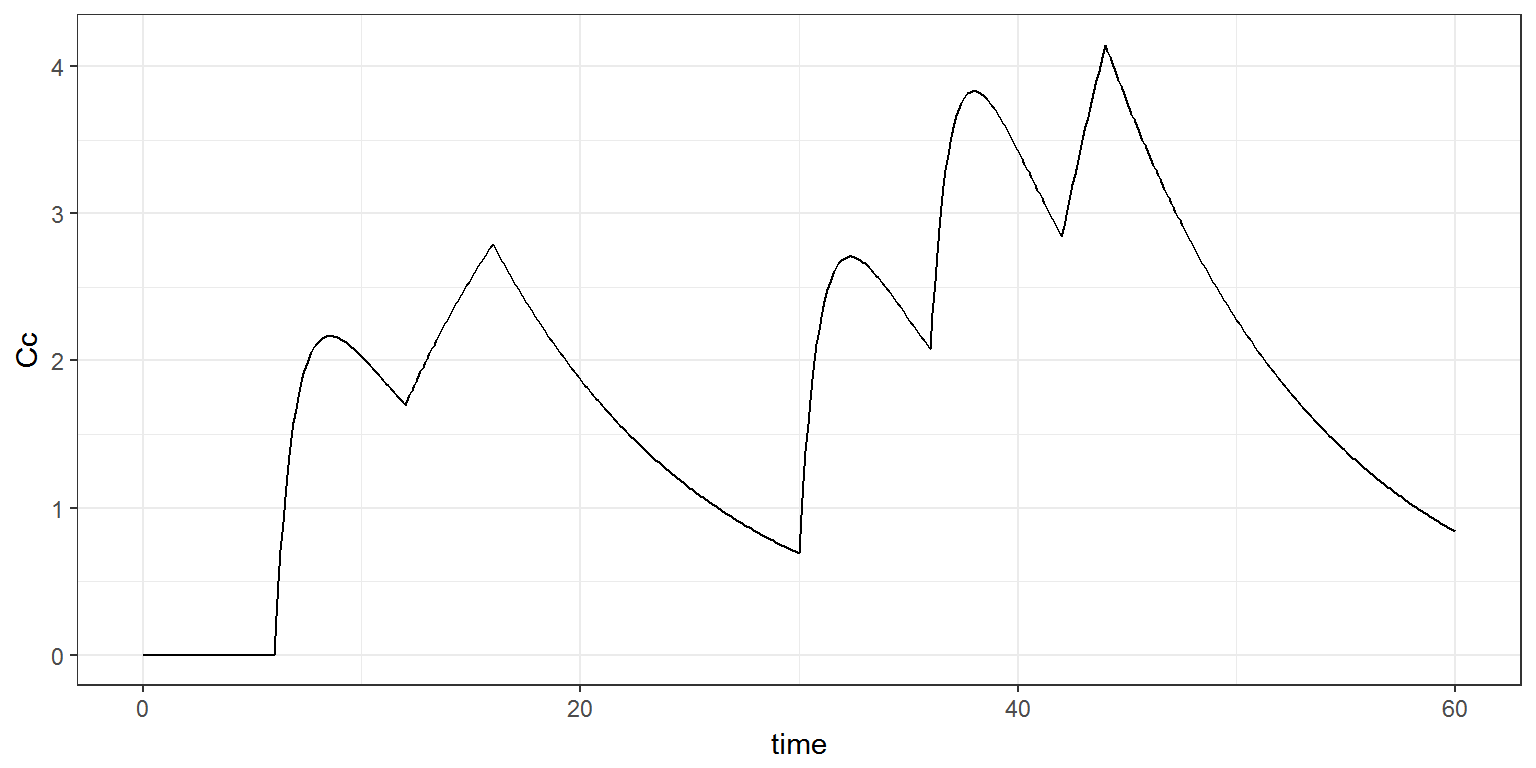Instead of defining the target compartments (i.e. the target components of the system) in the PK block of the Mlxtran code, it is possible to define them in the R code. Here, the Mlxtran pk2b.txt code has no block PK: [LONGITUDINAL] input = {ka, V, k} EQUATION: ddt_Ad = -ka*Ad ddt_Ac = ka*Ad - k*Ac Cc = Ac/V  The target components are defined as additional fields of adm1 and adm2. adm1 <- list(target = "Ad", time = c(6, 30, 36), amount = 40*p[["F"]]) adm2 <- list(target = "Ac", time = c(12,42), amount = 20, rate = c(5, 10)) res <- simulx(model = "model/pk2b.txt", parameter = p, output = Cc, treatment = list(adm1, adm2)) print(ggplot(data=res$Cc, aes(x=time, y=Cc)) + geom_line())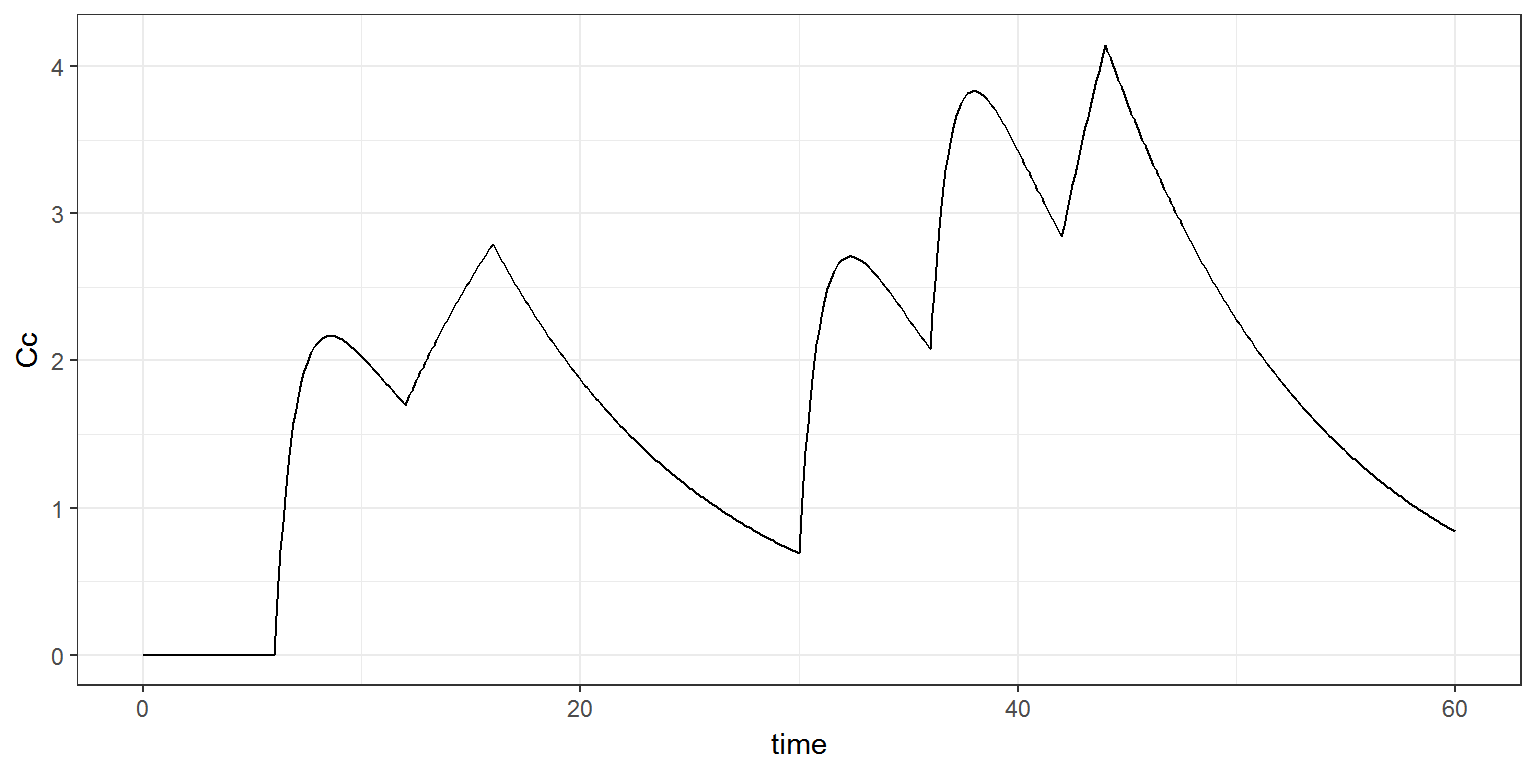## 2.2 Example 2

In this example, one type of dose is administered orally (adm=1) and absorbed into a latent compartment following a first-order absorption process, a second type is administered orally (adm=2) and absorbed into the central compartment following a zero-order absorption process, and a third type is directly administered intravenously to the central compartment (adm=3).

There is linear transfer from the latent to the central compartment. A peripheral compartment is linked to the central compartment. The drug is eliminated by a linear process from the latent compartment and a nonlinear process from the central one. Here, $$A_l$$ and $$A_c$$ are the drug amounts in the latent and central compartments.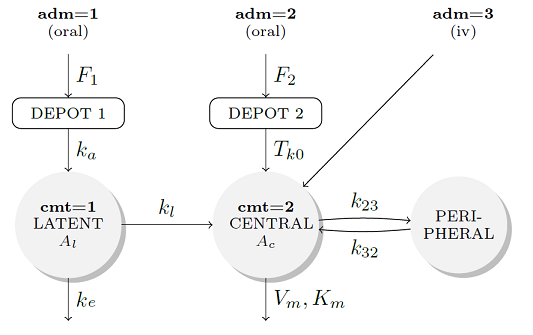This model is implemented in pk3.txt using PK macros:

[LONGITUDINAL]
input = {F1, F2, ka, Tk0, kl, k23, k32, V, k, Vm, Km}

PK:
compartment(cmt=1, amount=Al)
compartment(cmt=2, amount=Ac)
peripheral(k23,k32)
oral(type=1, cmt=1, ka,  p=F1)
oral(type=2, cmt=2, Tk0, p=F2)
iv(type=3, cmt=2)
transfer(from=1, to=2, kt=kl)
elimination(cmt=1, k)
elimination(cmt=2, Km, Vm)
Cc = Ac/V


We can then combine these three types of administration by defining three lists adm1, adm2 and adm3.

adm1 <- list(type=1, time=seq(6, 66, by=8),  amount=2)
adm2 <- list(type=2, time=seq(9, 57, by=12), amount=1)
adm3 <- list(type=3, time=seq(12,60, by=12), amount=1,rate=0.2)

p <- c(F1=0.5, F2=0.8, ka=0.5, Tk0=4, kl=0.5, k23=0.3,
k32=0.5, V=10, k=0.2, Vm=0.5, Km=1)

Cc <- list(name = "Cc", time = seq(0,to=80,by=0.1))

res <- simulx( model     = "model/pk3.txt",
parameter = p,
output    = Cc,
treatment = list(adm1, adm2, adm3))

print(ggplot(data=res$Cc, aes(x=time, y=Cc)) + geom_line())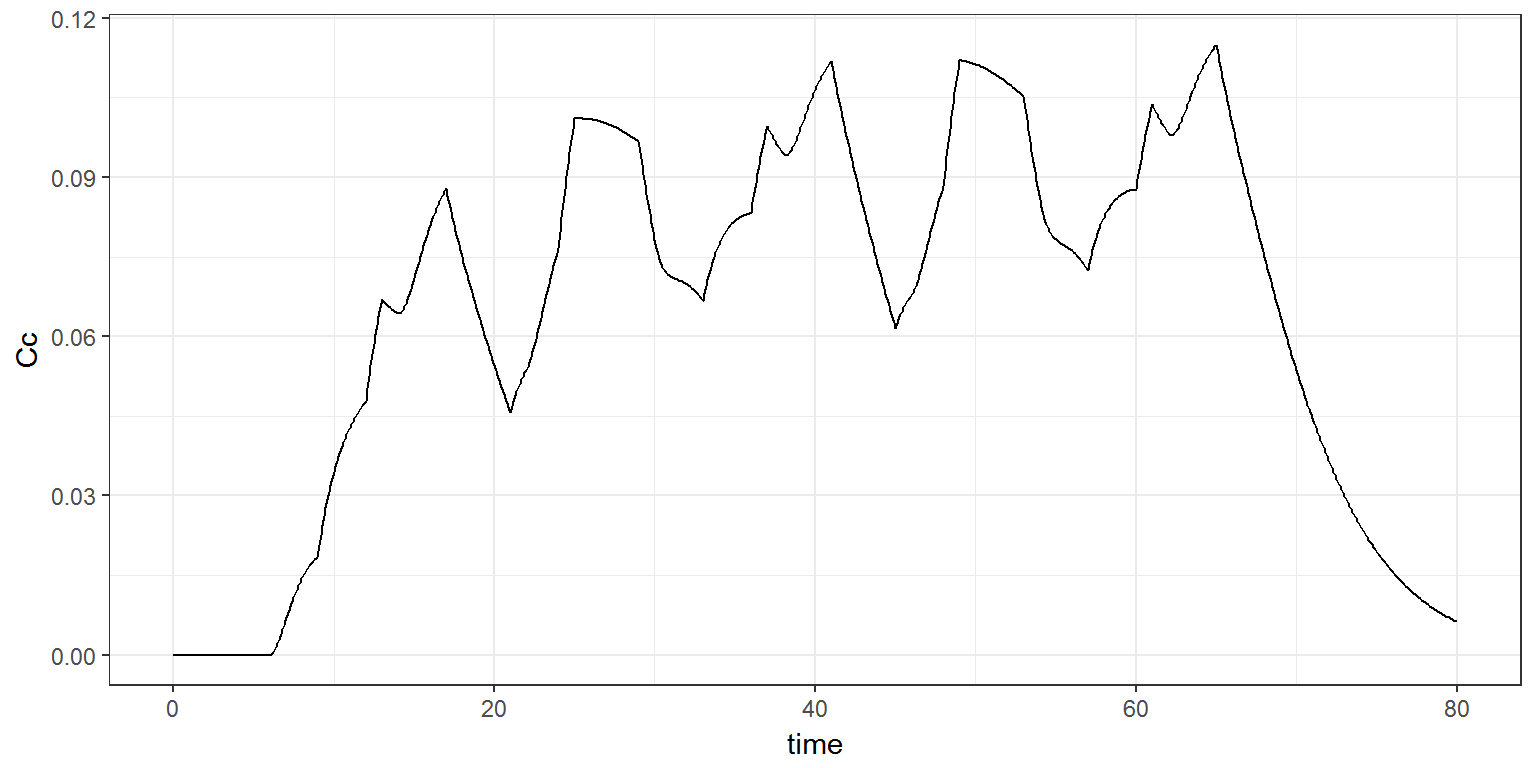Using the same model, we can also define different combinations of administration; In this example, will will consider a combination of adm1 and adm3 and a combination of adm2 and adm3. g1 <- list(treatment=list(adm1, adm3)) g2 <- list(treatment=list(adm2, adm3)) res <- simulx( model = "model/pk3.txt", parameter = p, output = Cc, group = list(g1, g2)) print(ggplot(data=res$Cc) + geom_line(aes(x=time, y=Cc, colour=id)) +
theme(legend.position=c(.95, .85)))